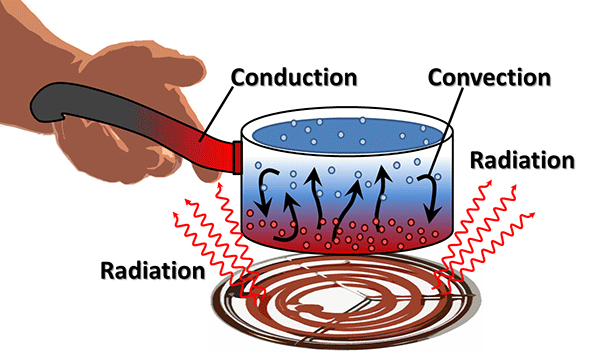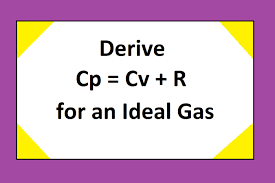# Heat Capacity - Relation between Cp and Cv

## Difference Between Cp and Cv

Introduction

Touch an engine after a ride, and it feels hot. Dip your finger in ice-water, and it feels cold. That's a no-brainer! But what if a polar bear, used to freezing Antarctic temperatures, touched the same things? Both might feel hot to a polar bear because it lives in much colder climatic conditions than we do. Hot and cold are correlative terms that can be used to compare how objects feel when they have more or less of a certain kind of energy, we call heat. What is this heat, where does it come from, and how does it move around our world? Let's explore more!

Heat

Heat energy is called Heat. When an object is very hot, it generally possesses a lot of heat energy; similarly, when the object is cold, it has less heat energy. The things which seem colder such as polar bears and icebergs have rather more heat energy than you. Every object consists of atoms, and molecules these molecules collide with each other like people in the crowd, and this is known as kinetic energy of matter.The Kinetic energy theory helps us to understand what will happen to energy when we heat something. If you put a vessel full of water on a hot stove, you are going to make the molecules in the water move around more quickly and collide with each other. The more heat we supply, the molecules move faster, and these molecules start moving apart. Eventually, they collide around so much that they break apart from one another. At that point, the liquid you have been heating turns into a gas, your water becomes vapor and starts evaporating away. The heat can be transferred from one place to another in different ways:

•Conduction
•Convection
• Radiation

Heat capacity

When the body absorbs the heat present, there is an increase in the temperature of the body. And when there is no heat, the temperature of the body decreases. The temperature of an object is the measure by the sum of the kinetic energy of the particles which makes the object. So when heat is received by an object this heat gets transformed into the kinetic energy of the particles and as a result of that temperature increases. Thus, the change in temperature is proportionate to the heat transfer.
The volume of heat supplied to heat an object can be expressed as:

Q = C dt

where,

Q = amount of heat supplied (J, Btu)

The C= heat capacity of the system or object (J/K, Btu/ oF)

dt = temperature rise (K, °C, oF)

J/K (joule per kelvin) is the SI unit of heat capacity. In the system of English, the units are British thermal units per pound per degree Fahrenheit (Btu/oF).

Specific heat capacity

The Specific Heat Capacity is the amount of heat required to change the temperature of a mass unit of an element by one degree. Specific heat is a more general term for the same.

The heat provided to a mass can be expressed as:

dQ = m c dt

There are two determinations of Specific Heat for gases and vapor:

Cp = (δh / δT) p - The specific Heat at constant pressure

Cv = (δh / δT)v - The specific Heat at constant volume

Gas Constant: It is denoted by R, can be expressed as:

R = CP – Cv

The ratio of Specific Heat: It is denoted by k, can be expressed as

K = CP/CV

The Molar Heat Capacity

The molar heat capacity is the amount of heat required to increase the temperature of one mole of a substance by one degree at constant pressure and volume. The molar heat capacity is expressed in the form of joules per moles per degrees Kelvin (or Celsius)

The formula q = n C ∆T represents the heat required q to bring about the change in the ∆T variation in temperature of one mole of any kind of matter. C is the constant here it is called the molar heat capacity of the body. Thus, the capacity of the molar heat of any substance is determined as the amount of heat energy needed to change the temperature of 1 mole of that element by 1 unit. It depends on the type, quantity, and composition of the system.

There are two types of the molar heat capacity, that is, CPand CV

What is CP?

The molar heat capacity C of an object is the amount of heat needed to increase the temperature of one mole of an element by one degree at constant pressure is called the Cp(constant pressure).

What is a CV?

The molar heat capacity C of an object is the amount of heat needed to increase the temperature of one mole of an element by one degree at constant volume is called the CV (constant volume).

The relationship between the heat capacity of CP and Cv.

The molar heat capacity at constant pressure Cpis always greater than the heat capacity at constant volume CV, because when heat is added to the constant pressure, there is always an expansion in the substances.

Derivation of Cp and Cv relation using ideal gasesFrom the equation q = n C ∆T, we can get,
At constant pressure CP, we get
qP = n CP∆T
This value is exactly the same as the change in enthalpy, that is,
qP = n CP∆T = ∆H
which is also the same in the constant volume CV, we get
qV = n CV∆T
This value is exactly equal to the change in internal energy, that is,
qV = n CV∆T = ∆U
We know that by the molar heat capacity that one mole (n=1) of an ideal gas,
∆H = ∆U + ∆(pV ) = ∆U + ∆(R * T) = ∆U + R * ∆T
Therefore, ∆H = ∆U + R ∆T
By substituting the values of ∆H and ∆U from the above equation, we will get
CP∆T = CV∆T + R ∆T
CP = CV + R
CP – CV = R

Hence it is proved that the Cp is always greater than the Cv

The Difference between the Cp and Cv

 Cv Cp Cp is the amount of capacity of the heat energy that an element absorbs or release with the change the temperature where a volume change does not happenVolume remains the same, that is, constantAssociated to the internal energy of a system Cv is the amount of capacity of the heat energy that an element absorbs or release within the change the temperature where a pressure change does not happenPressure remains the same, that is, constantAssociated to the enthalpy of a system

Answer the following questions

• 1. What is heat capacity and write its formula?

• 2. Explain the different ways in which heat energy can be transferred from one to another place?

• 3. How to do get to know that whether the substance opposes heat capacity or not?

• 4. What do you mean by the specific heat capacity and write its formula?

• 5. Write the formula to show gas constant?

• 6. State the differences between the Cp and Cv?

• Fill in the blanks

• 1. The temperature of an object is the measure by the sum of the ____________of the particles which makes the object (Ans: kinetic energy)

• 2. R=______________ (Ans:CP – Cv)

• 3. The molar heat capacity C of an object is the amount of heat needed to increase the temperature of one mole of an element by one degree at ______________is called the Cp (Ans:constant pressure)

• 4. The molar heat capacity at constant pressure Cpis always ________________the heat capacity at constant volume CV (Ans:greater than)